## [ITOC-ch7] TIME COMPLEXITY

### 7.1 MEASURING COMPLEXITY

BIG-O AND SMALL-O NOTATION

$f(n) = O(g(n)) \ \Longleftrightarrow f(n) \ \le cg(n)$

$f(n) = o(g(n)) \ \Longleftrightarrow f(n) \ \lt cg(n)$

polynomial bounds: with the form $n^c$

exponential bounds: with the form $2^{ ( n^{\delta} ) }$

Time complexity

• M is deterministic
• f(n) is the maximum number of steps that M uses on any input of length n.
•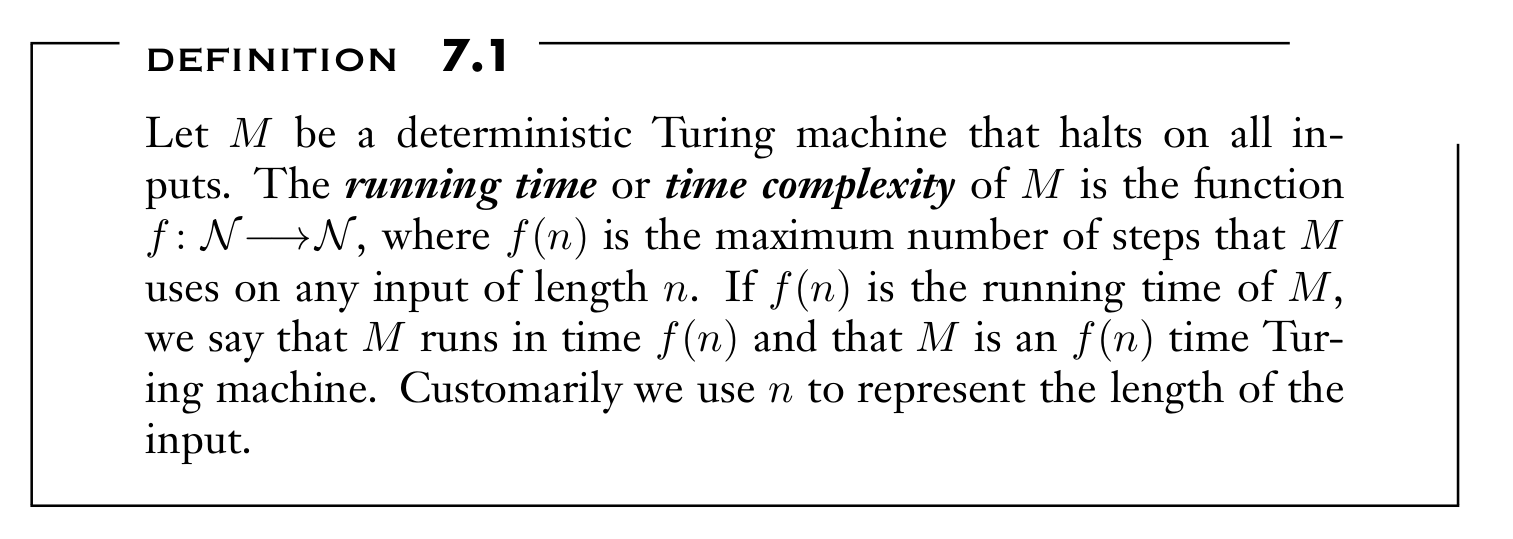ANALYZING ALGORITHMS

TIME(t(n))

• collection of languages that are decidable by a TM
•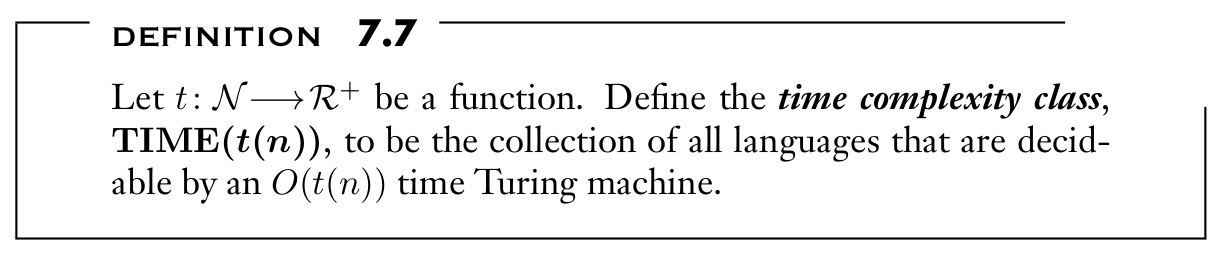nondeterministic Time complexity

• N is nondeterministic
• f(n) is the maximum number of steps that N uses on any branch of its computation on any input of length n
•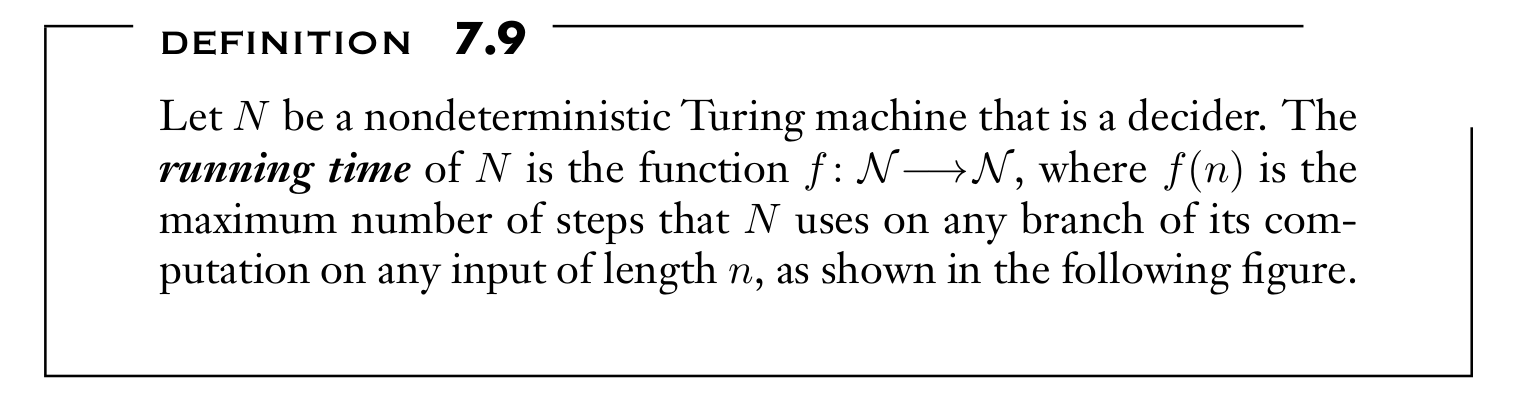NTIME(t(n))

• collection of languages that are decidable by a NTM
•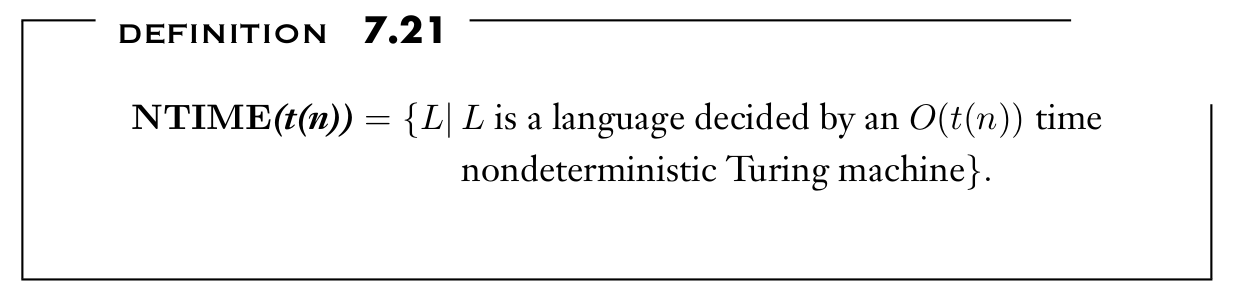COMPLEXITY RELATIONSHIPS AMONG MODELS

computational model can affect the time complexity of languages

Theorem 7.8

• Let $t(n)$ be a function, where $t(n) ≥ n$. Then every $t(n)$ time multitape Turing machine has an equivalent $O(t^2 (n))$ time single-tape Turing machine
• at most polynomial difference

Theorem 7.11

• Let $t(n)$ be a function, where $t(n) ≥ n$. Then every $t(n)$ time nondeterministic single-tape Turing machine has an equivalent $2^{O(t(n))}$ time deterministic single-tape Turing machine.
• at most exponential difference

### 7.2 THE CLASS P

POLYNOMIAL TIME

polynomially equivalent

• one of them can simulate another with only a polynomial increase in running time
• All reasonable deterministic computational models are polynomially equivalent.

class P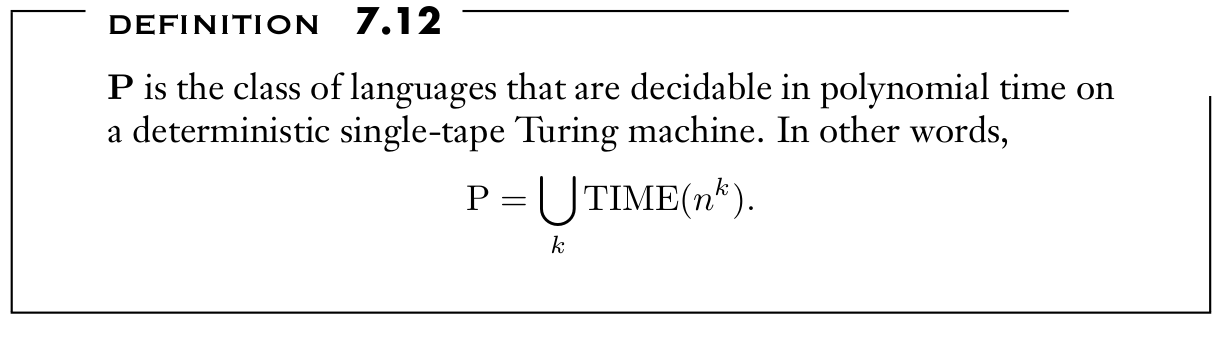Examples

• $PATH = \{ < G, s, t> | \ G \ is \ a \ directed \ graph \ that \ has \ a \ directed \ path \ from \ s \ to \ t\}$
• $RELPRIME = \{ < x, y>| \ x \ and \ y \ are \ relatively \ prime\}$
• Every context-free language is a member of P.

### 7.3 THE CLASS NP

class NP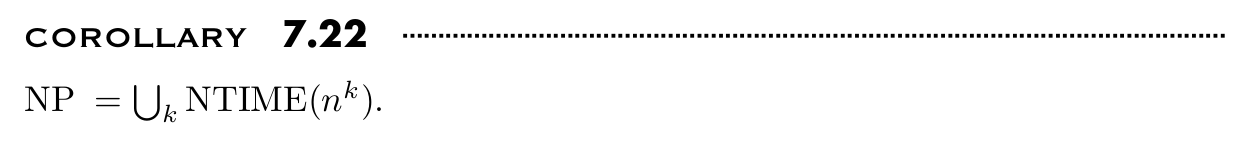Another equivalent definition

• A verifier for a language A is an algorithm V , where
$A = \{ w | \ V \ accepts \ < w, c > \ for \ some \ string \ c\}$
• certificate: additional information $c$ the verifier use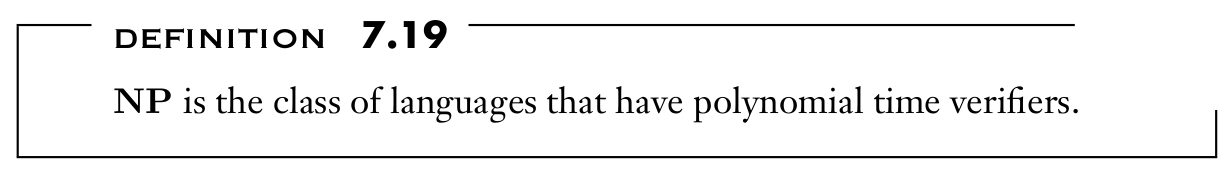Proof of equivalence

Theorem 7.20

• A language have polynomial verifiers iff it is decided by some nondeterministic polynomial time Turing machine.

Proof

1. Assume that A is verified by a polynomial time TM $V$ and construct $N$
• N nondeterministically select string c and run V for the verification
2. Assume that A is decided by a polynomial time NTM $N$ and construct $V$
• V treat each symbol of c as a description of the nondeterministic choice

Examples

• $HAMPATH = \{ < G, s, t >| \ G \ is \ a \ directed \ graph \ with \ a \ Hamiltonian \ path \ from \ s \ to \ t\}$
• $COMPOSITES = \{x| \ x = pq, \ for \ integers \ p, q > 1 \}$
• $CLIQUE = \{ < G, k>| \ G \ is \ an \ undirected \ graph \ with \ a \ k-clique \}$
• $SUBSET-SUM = \{ < S, t>| \ S = \{x_1 , . . . , x_k \}, and \ for \ some \ \{y_1 , . . . , y_l \} ⊆ \{x_1 , . . . , x_k \}, \ we \ have \ Σy_i = t\}$

co-NP

• languages that are complements of languages in NP
• Verifying that something is not present seems to be more difficult than verifying that it is present.

THE P VERSUS NP QUESTION

$P \subset NP$ or $P = NP$

### 7.4 NP-COMPLETENESS

POLYNOMIAL TIME REDUCIBILITY

• define a version of reducibility that takes the efficiency of computation into account

• the reduction function is polynomial time computable

• $A ≤_P B$ than A is no harder than B

DEFINITION OF NP-COMPLETENESS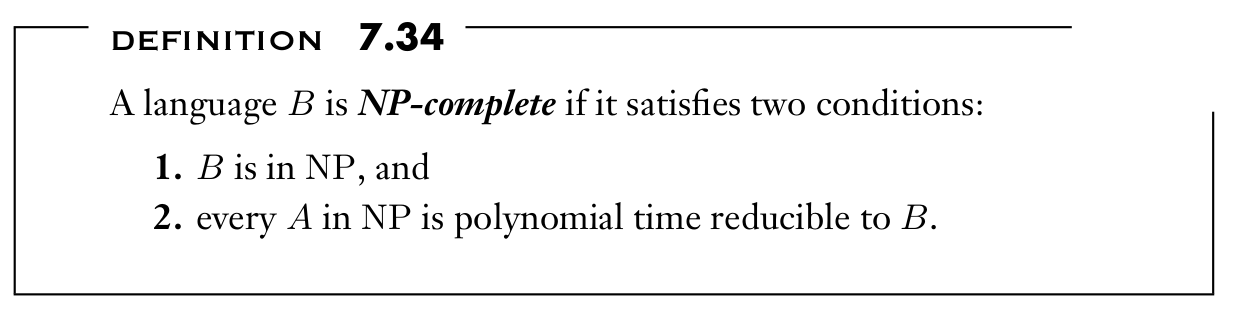Theorem 7.35

• If $B$ is NP-complete and $B ∈ P$, then $P = NP$.

Theorem 7.36

• If $B$ is NP-complete and $B ≤_P C$ for $C$ in $NP$, then $C$ is NP-complete.

THE COOK—LEVIN THEOREM

$SAT$ is NP-complete.

Idea

• By DEF: show that every language $A$ in NP is polynomial time reducible to SAT
• Reduction function: takes a string w and produces a Boolean formula φ that simulates the NP machine for A on input w, while w is in A iff φ is satisfiable
• the problem of determining whether N accepts w is equivalent to the problem of determining whether an accepting tableau for N on w exists.
• 将每个configuration里的每个symbol都设为一个变量, 按照A accept w的过程来模拟布尔表达式, 构造的表达式只保证符合TM的计算过程(对应的表格是合法的), 只有当accept的时候表达式才有真的赋值

Proof

• variable: For each $i$ and $j$ between 1 and $n^k$ and for each $s$ in $C = Q ∪ Γ ∪ \{ \# \}$, we have a variable, $x_{i,j,s}$, If $x_{i,j,s}$ takes on the value 1, it means that $cell [i, j]$ in the tableau contains an s

• formula φ: $φ{cell} ∧ φ{start} ∧ φ{move} ∧ φ{accept}$

• $φ_{cell} ​$: ensure that there must have exactly one variable on for every cell (tableau is valid)• $φ_{start}$: ensure that the first row of the table is the starting configuration• $φ_{accept}$:ensure that an accepting configuration occurs in the tableau• $φ_{move}$: ensure that each row’s configuration legally follows the preceding row’s configuration according to N ’s rules.

• Ensure each 2 × 3 window of cells is legal• complexity of the reduction: $O(n^{2k} )$

### 7.5 ADDITIONAL NP-COMPLETE PROBLEMS

(几乎都是从3SAT reduce 来的….感觉比CLRS难哭唧唧感觉还是CLRS讲的懂一点x)

CLIQUE

VERTEX-COVER

HAMPATH

UHAMPATH

SUBSET-SUM